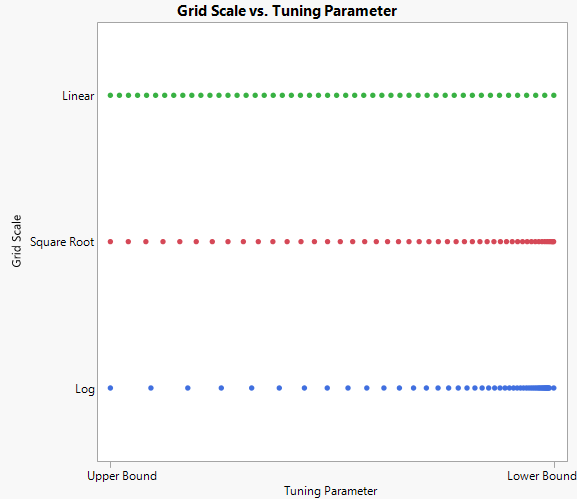Publication date: 08/13/2020

##Statistical Details for Advanced Controls

###Grid

The tuning parameters for ridge regression and the Lasso that best minimize the penalized likelihood are found by searching a grid of tuning parameter values. This grid of values lies between a lower and an upper bound for the tuning parameter. You can specify the number of grid points under Advanced Controls.

The lower bound is zero except in special cases where it is set to 0.0001. See Tuning Parameter. When the lower bound for the tuning parameter is zero, the solution is unpenalized and the coefficients are the MLEs. The upper bound is the smallest value for which all of the non-intercept terms are zero.

The grid of values between the lower and upper bounds is iteratively searched to determine the best value of the tuning parameter. The grid of possible tuning parameters can be set up in three different scales: linear, log, and square root. The default grid scale is square root.

In some cases, there is a large gap between the unpenalized estimates and the previous step. This large gap can distort the solution path. The log scale focuses its search on small tuning parameter values with few large values, whereas the linear scale evenly disperses the search from the minimum to the maximum value. The square root scale is a compromise between the other two scales. Figure 6.7 shows the different grid scales.

Figure 6.7 Options for Tuning Parameter Grid Scale